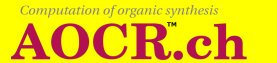# 1. Organic chemistry basics

### 1.2. Reactions

In an organic reaction the starting compounds A1 ... An transform into the compounds B1 ... Bm, whose symbols, charges and electrons are the same as in A1 ... An and occur exactly as often as in A1 ... An.

Any combination of the compounds B1, ..., Bm is produced by or obtained from or synthesized in the reaction.

An example of an organic reaction, the aldol condensation, is given in Figure 1.2. Here the upper formula of two compounds transforms into the lower formula of two compounds.

Figure 1.2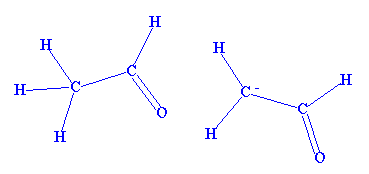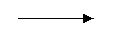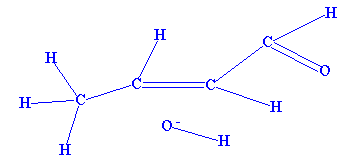Figure 1.3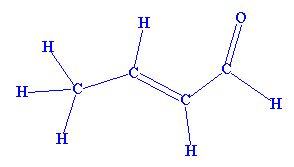A compound obtained from the reaction in the above example of Figure 1.2, is crotonaldehyde which is represented in Figure 1.3.